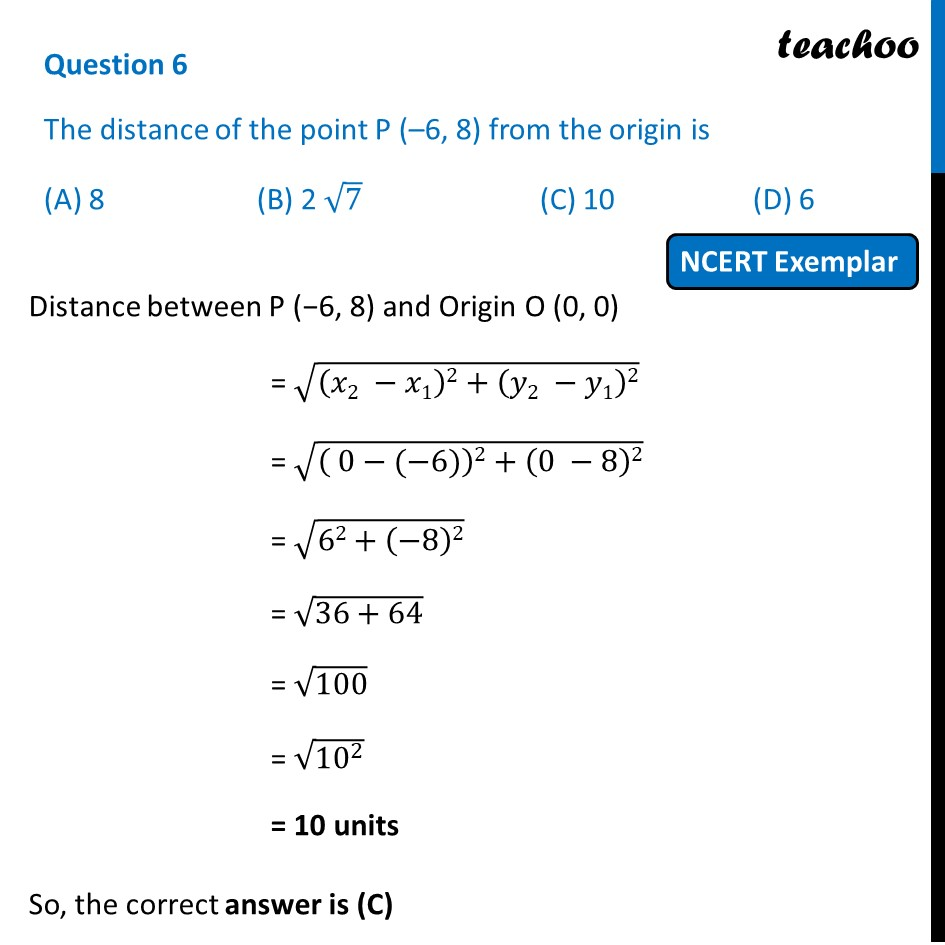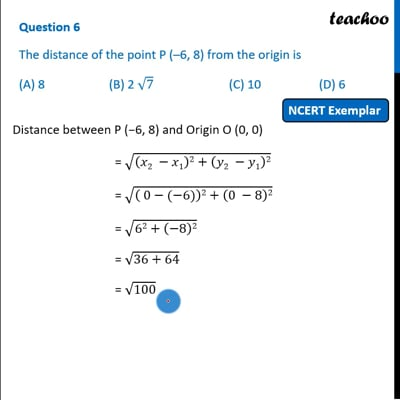NCERT Exemplar - MCQ

Chapter 7 Class 10 Coordinate Geometry (Term 1)
Serial order wise

## (A) 8   (B) 2 √7   (C) 10   (D) 6This video is only available for Teachoo black users

### Transcript

Question 6 The distance of the point P (–6, 8) from the origin is (A) 8 (B) 2 √7 (C) 10 (D) 6 Distance between P (−6, 8) and Origin O (0, 0) = √((𝑥2 −𝑥1)2+(𝑦2 −𝑦1)2) = √(( 0−(−6))2+(0 −8)2) = √(62+(−8)2) = √(36+64) = √100 = √(10^2 ) = 10 units So, the correct answer is (C)